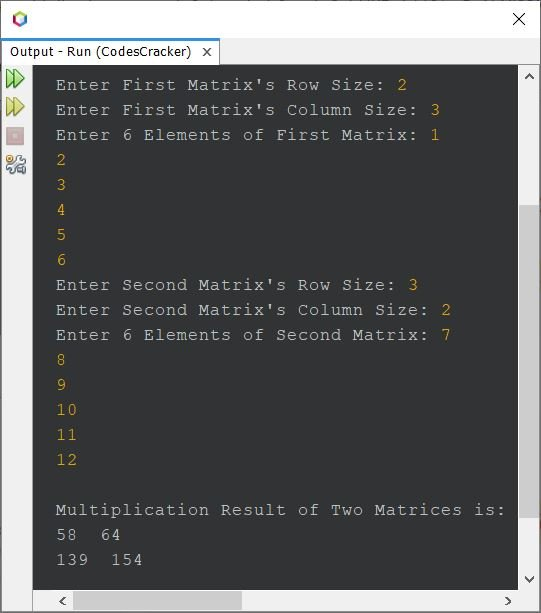# Java Program to Multiply Two Matrices

This article covers a program in Java that find and prints the multiplication result of any two matrices, entered by user at run-time of the program. The program is created in following two ways:

• Multiply two 3*3 given matrices
• Multiply two matrices of given order and elements

## Multiply Two 3*3 Given Matrices in Java

The question is, write a Java program to perform matrix multiplication based on user input. The program given below is its answer:

```import java.util.Scanner;

public class CodesCracker
{
public static void main(String[] args)
{
int i, j, k, sum=0;
int[][] matrixOne = new int;
int[][] matrixTwo = new int;
int[][] matrixThree = new int;
Scanner scan = new Scanner(System.in);

System.out.print("Enter 9 Elements of First Matrix: ");
for(i=0; i<3; i++)
{
for(j=0; j<3; j++)
{
matrixOne[i][j] = scan.nextInt();
}
}
System.out.print("Enter 9 Elements of Second Matrix: ");
for(i=0; i<3; i++)
{
for(j=0; j<3; j++)
{
matrixTwo[i][j] = scan.nextInt();
}
}

// multiplying the two matrices
for(i=0; i<3; i++)
{
for(j=0; j<3; j++)
{
sum = 0;
for(k=0; k<3; k++)
{
sum = sum + (matrixOne[i][k] * matrixTwo[k][j]);
}
matrixThree[i][j] = sum;
}
}

System.out.println("\nMultiplication Result of Two Matrices is:");
for(i=0; i<3; i++)
{
for(j=0; j<3; j++)
{
System.out.print(matrixThree[i][j]+ "  ");
}
System.out.print("\n");
}
}
}```

The snapshot given below shows the sample run of above program with user input 1, 2, 3, 4, 5, 6, 7, 8, 9 as nine elements for first, whereas 1, 2, 1, 2, 4, 6, 7, 2, 5 as nine elements for the second matrix:## Multiply Two Matrices of Given Order and Elements in Java

This program is basically the modified version of previous program. As this programs works to multiply 3*3 matrices as well as any other matrix of any order, entered by user at run-time:

```import java.util.Scanner;

public class CodesCracker
{
public static void main(String[] args)
{
int i, j, k, sum=0, rOne, cOne, rTwo, cTwo;
Scanner scan = new Scanner(System.in);

System.out.print("Enter First Matrix's Row Size: ");
rOne = scan.nextInt();
System.out.print("Enter First Matrix's Column Size: ");
cOne = scan.nextInt();
int[][] matrixOne = new int[rOne][cOne];
System.out.print("Enter " +(rOne*cOne)+" Elements of First Matrix: ");
for(i=0; i<rOne; i++)
{
for(j=0; j<cOne; j++)
matrixOne[i][j] = scan.nextInt();
}
System.out.print("Enter Second Matrix's Row Size: ");
rTwo = scan.nextInt();
if(cOne != rTwo)
{
System.out.println("\nMultiplication not possible!");
return;
}
System.out.print("Enter Second Matrix's Column Size: ");
cTwo = scan.nextInt();
int[][] matrixTwo = new int[rTwo][cTwo];
System.out.print("Enter " +(rTwo*cTwo)+" Elements of Second Matrix: ");
for(i=0; i<rTwo; i++)
{
for(j=0; j<cTwo; j++)
matrixTwo[i][j] = scan.nextInt();
}

int[][] matrixThree = new int[rOne][cTwo];

// multiplying the two matrices
for(i=0; i<rOne; i++)
{
for(j=0; j<cTwo; j++)
{
sum = 0;
for(k=0; k<cOne; k++)
sum = sum + (matrixOne[i][k] * matrixTwo[k][j]);
matrixThree[i][j] = sum;
}
}

System.out.println("\nMultiplication Result of Two Matrices is:");
for(i=0; i<rOne; i++)
{
for(j=0; j<cTwo; j++)
System.out.print(matrixThree[i][j]+ "  ");
System.out.println();
}
}
}```

The sample run of above Java program, on multiplying two matrices of given order and elements, is shown in the snapshot given below:#### Same Program in Other Languages

Java Online Test

« Previous Program Next Program »